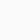# Servomotor + gearbox + Linear actuatorTo determine the linear distance traveled by a system consisting of a servomotor connected to a gearbox and a linear actuator, you’ll need to consider several factors:

1. Gear Ratio (GR): The gear ratio represents the relationship between the input (motor) shaft and the output (linear actuator) shaft. It indicates how many revolutions of the motor shaft are required to make one revolution of the linear actuator shaft.
2. Linear Travel per Revolution (LTR): This is the linear distance the linear actuator moves with one complete revolution of its shaft. It’s determined by the mechanical design of the actuator, specifically the screw or mechanism used to convert rotary motion into linear motion.
3. Motor Steps per Revolution: If your servomotor is a stepper motor, it moves in discrete steps. The number of steps per motor revolution depends on the motor’s specifications.

To calculate the linear distance traveled for one revolution of the motor shaft, you can use the following formula:

plaintext
`Linear Distance = GR * LTR`

Where:

• `Linear Distance` is the linear distance traveled by the linear actuator for one revolution of the motor shaft.
• `GR` is the gear ratio, representing the reduction or multiplication factor between the motor and linear actuator.
• `LTR` is the linear travel per revolution of the linear actuator.

For example, if you have a gear ratio of 5:1 (motor shaft rotates 5 times for one rotation of the linear actuator shaft) and the linear actuator travels 2 inches per revolution, the linear distance traveled for one motor revolution would be:

plaintext
`Linear Distance = 5 * 2 inches = 10 inches`

So, for each revolution of the motor shaft, the linear actuator will travel 10 inches.

If you are using a servo motor (which can rotate continuously) rather than a stepper motor (which moves in discrete steps), you can calculate the linear distance based on the motor’s specifications and the mechanical setup, as described above. Additionally, consider any feedback devices like encoders that might provide accurate position information for your specific system.

### mapping robot### Circuit to turn a servo with 2 inputs